### Spectral estimation of what ?

There are two totally different situations in which one would like to obtain the spectrum of a signal: the first is an estimation of the Fourier transform of a given (piece of) continuous-time signal, as a time-dependent, but "deterministic" function.  The second, which is what we are concerned mainly, is the estimation of the spectral power density of an ensemble of time-dependent continuous signals.  A basic error consists in confusing both.  Nevertheless, before understanding spectral estimation of ensembles, it is necessary to understand the spectral estimation of the Fourier transform of a given signal.  Therefore this contribution talks about the estimation of the Fourier transform of a given deterministic signal and not of the spectral estimation of an ensemble.

In both cases, spectral estimation is done by using time-limited "pieces of signal" and using a sampling at a finite sampling frequency.   This will introduce some effects on the estimated spectrum: aliasing and windowing.

### Aliasing

Aliasing is a consequence of the finite sample frequency, and is described by Nyquist's theorem: a sampling frequency of 2 fs divides the spectrum into successive bands of width fs and maps these bands on one another in the direct sense or in the flipped-over sense.  Usually, Nyquist's theorem is formulated in the sense that the maximum frequency of the sampled signal is fs.  But in fact, sampling makes equivalent all frequencies f which differ by 2 fs, or which differ by 2 fs with -f.

If the sample frequency is 1 KHz, then a signal which is a sine wave of 10 Hz will be equivalent to a signal of 1010 Hz or a signal of 990 Hz.  A sine wave of 490 Hz will be equivalent to a sine wave of 510 Hz.  The piece from 500 Hz to 1 KHz is mapped onto the 0 - 500 Hz range, but flipped-over.  The piece from 1000 Hz to 1500 Hz is mapped onto the 0 - 500 Hz piece in the direct sense, the piece from 1500 Hz to 2000 Hz is mapped onto the 0 - 500 Hz part, but flipped over.  And so on.  To be more precise,

`sin(2 π 10 t), - sin(2π 990 t), sin(2 π 1010 t) , ...  `

have the same sample values when sampled every 1 ms.

Note that this equivalence in sampling is independent of what one is going to do with the samples, such as do spectral estimation, filtering or what ever: it is the nature of the sampling itself that gives rise to this equivalence.

If a frequency of 10 Hz and of 990 Hz cannot be distinguished from each other, that means that if we sample a signal that contains both frequencies, it will not be possible after the fact to say which part came from the 10 Hz component and which part came from the 990 Hz component: the samples of both components will be added together.  It will not be possible to restore the original signal from these samples.  However, Nyquist's theorem tells us too, that if a signal is guaranteed to be confined in the band 0 - 500 Hz, there is only one continuous-time signal that corresponds to the samples ; as such, the continuous-time signal can be restored, and no information is lost.  Most of the time one talks about the base band (that is the band from 0 Hz to fs), but this is in fact not necessary.  If one knows that the continuous-time signal is confined to ANY band from n. fs to (n+1) fs , and one knows which band it is, then this signal can be restored.

For instance, if one has a signal around 400 MHz, with a bandwidth of 100 KHz, in principle one can sample such a high-frequency signal with a 200 KHz sampling frequency, at least if the signal falls nicely within one band (say, from 400 MHz to 400.1 MHz), which is the 4000th harmonic band.  This is of course great news: a sample rate of 200 KHz gives a data flux which can easily be treated by most digital systems, while a data rate of 800 MHz (which would be necessary to sample directly the incoming sample 'naively' in the base band) would swamp but the most powerful digital systems.

But there is a caveat of course: one needs to sample of course the 400 MHz signal with a sufficient precision (about a nanosecond or less) as if it were in the base band, even though one only needs one sample every 5 microseconds.  Most digitizers that run at 200 KHz can only sample a signal with a precision which will be of the order of a microsecond or so.  In fact, one should use a digitizer that can run at 800 MHz in a certain way, but only keep one sample every 5 microseconds (one every 4000 samples.   Some digitizers are able to sample much more precisely than their shortest sample period.

### Anti-aliasing filter

As we saw, aliasing is a phenomenon, inherent to the operation of sampling at a finite frequency and independent of what we want to do with those samples afterwards.  It has nothing to do per se with spectral estimation but is a universal phenomenon of confusion between different continuous-time signals which produce identical samples.  If we want to avoid this confusion, then an analogue anti-aliasing filter is absolutely necessary unless we are guaranteed that only signals within one allowed band will be present (which we can't, from the moment that some broad band noise is present, which is almost always the case).

Most of the time, when talking about an anti-aliasing filter, one thinks of a low-pass filter that cuts off at frequency fs but as we saw, in fact, any higher-order band from n fs to (n+1) fs is possible.  In that case, the anti-aliasing filter has to be a band-pass filter with these frequencies as cut-off frequencies.

In practice it is hard to build a highly selective filter and it is better to use a somewhat higher sample rate than strictly necessary, in order to make a low pass or band pass filter that cuts off at the useful band, and has some roll-off space until the actual digital bandwidth is reached.

For instance, if we have a useful signal in the band 0 - 30 KHz, then strictly speaking, a 60 KHz sampling rate would be sufficient.  However, it would imply a perfect low pass filter, which has to let through of course all the useful signal in the band 0 - 30 KHz, and would have to cut away perfectly the band 30 KHz - 60 KHz which would otherwise be folded into the samples.  A noise signal at 31 KHz would mimic as a 29 KHz noise signal if the filter doesn't cut off strongly at 31 KHz.

This is why it is much easier to sample at, say, 100 KHz, which puts the digital bandwidth at 50 KHz.  Now, we only need a filter that lets the band 0 - 30 KHz pass, and needs to cut off strongly only above 50 KHz.  Our noise signal at 31 KHz will appear as a genuine 31 KHz signal in the digital domain, and will not be confused with the 29 KHz within the useful band.  A 51 KHz signal should be, on one hand, sufficiently repressed by the filter, but also, it will mimic as a 49 KHz signal, which is still outside of the useful band.  It is only a signal of 71 KHz, even more suppressed by the filter, that will mimic as a 29 KHz signal within the useful band.  As such, increasing somewhat the sampling rate has given enough roll off room (essentially between 30 KHz and 70 KHz) to allow for a sufficiently simple and realisable filter to be built.

### Windowing and leakage

If we have a finite piece of signal in time, say from 0 to T, and zero outside this window, then:

1. the Fourier transform of this signal is continuous
2. the spectral bandwidth is infinite

This makes, strictly speaking, finite pieces of signal incompatible with limited bandwidth requirements of anti-aliasing.  Strictly speaking, one should stop here in the attempt to find any Fourier spectral representation of a finite piece of sampled signal, as it is a contradiction.  On the other hand, digitally, there is no other way.  Of course, this is partly due to the definition of the Fourier transform, which is a transform that applies to continuous, infinite-duration signals.  Of course one can find other transforms that are compatible with a finite set of sampled values, and these transforms exist and are used.  But we were after the actual estimation of the actual Fourier transform of, indeed, a continuous-time, infinite duration signal, remember ?  So there's no point in thinking of other transforms if this is the thing we wanted.  The only thing we learn in fact, is that, from a finite piece of sampled signal, we will not be able to recover the exact Fourier transform. But maybe we will be able to find some kind of approximation to it.

There's no way we drop the fact of having finite sampling, and a finite window in time, because this is the only way to obtain a result during our life times !

In a way it is perfectly normal that we cannot calculate exactly the Fourier spectrum of an infinitely long signal by only keeping a part of it, because the spectrum is influenced by all parts of the signal ; if we only have a part of it, then of course we ignore those other parts and we cannot calculate the right spectrum.

Somehow, there is a kind of hypothesis that the piece of signal we consider, is "representative" for the whole part that we ignore.   It is certainly not by setting it to zero and calculate the spectrum of that other signal, that is infinitely long, but is zero except for a finite window, that we have the best reconstruction of the original, infinitely long signal.  This is why the best way to "reconstruct" the infinitely long signal from our finite piece, is to consider this finite piece as repeated infinitely in the infinite-duration signal instead of putting that signal to zero outside of the window.

We illustrate this with a simple example: suppose that the original signal is a sine function (but we don't know it).  We take a finite piece of it, from 0 to 2 seconds, and put the rest to 0.  Then we repeat that piece (of course, to make a plot, we have to limit that plot in time too !  But let your mind's eye think of those plots as infinitely extended...)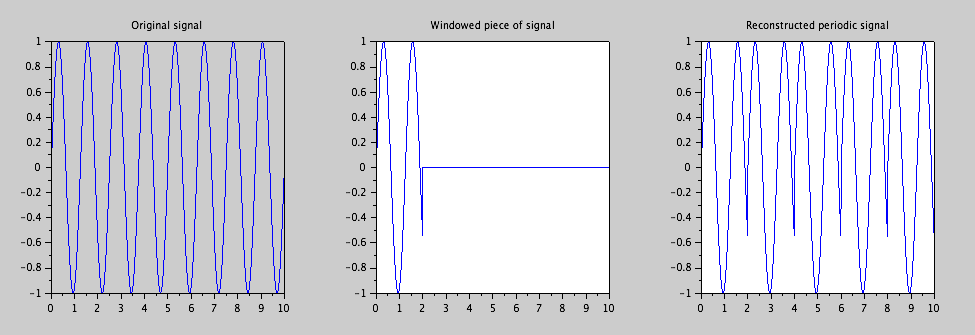As such, the new, hypothetical, reconstructed infinite-duration signal that we build in a hypothetical way from our finite piece of real signal has become a periodic signal.  And a periodic signal has only non-zero spectral content on a discrete grid, corresponding to multiples of the frequency Fw = 1/T.  In fact, there is a relationship between the continuous Fourier transform of the finite piece of signal, with zero value outside of the time window from 0 to T on one hand, and the discrete spectral lines of the periodic signal, made up by repeating that piece of signal indefinitely:

The amplitudes of discrete spectral lines at the frequencies which are integer multiples of Fw are proportional to the sample values of the continuous spectrum of the finite piece with zeros outside, at those frequencies. These amplitudes correspond to the coefficients of the Fourier series of that periodic signal.

We can illustrate that in the case of our sine signal at 0.8 Hz: the original Fourier spectrum was, in this particular example, just a single line (green).  The finite piece of sine had a continuous Fourier transform with infinite extend (blue).  The reconstructed periodic signal from this finite piece has a line spectrum (red) at multiples of Fw (here, 0.5 Hz because the window width is 2 s), and these lines are proportional to the sample values of the continuous spectrum of the finite piece.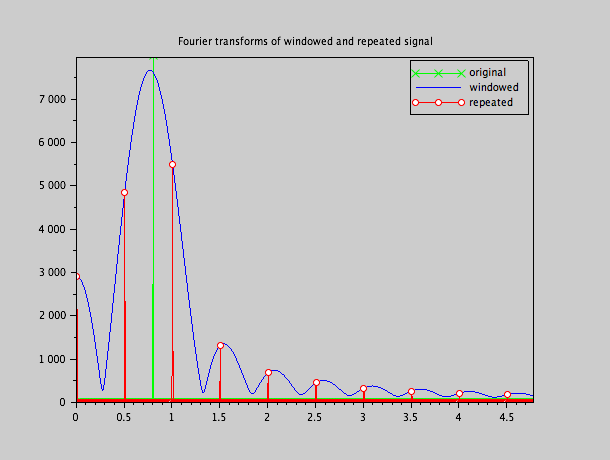In a certain way, repeating the sole piece of signal that we really have, indefinitely, is the best kind of guess of what was the whole signal. A periodic signal, however, can have a finite spectral content.  It doesn't have to, but it can, contrary to a finite piece of non-zero signal.   How is this possible ?  How can it be that the spectral lines of the repeated signal are proportional to the samples of the Fourier transform of the finite piece, which has to have infinite spectral content, while the repeated signal has only finite spectral content ?  The only possibility is that the continuous Fourier transform passes through zero for every high-order sample.  It means that the Fourier series of the periodic signal stops in its development, and only has a finite number of non-zero terms.  It means, in other words, that the periodic signal is made up of a finite sum of sine and cosine terms of which the frequencies are multiples of Fw, with no higher frequency than fs.  In that case, and only in that case, will the sampling over a finite time T at a sample frequency fs allow us a correct estimation of the Fourier transform of a signal.

When one limits an infinite-duration signal to a finite piece between 0 and T, and one sets the rest to 0, this can be seen as the multiplication of the original signal with a window function which is 0 everywhere, except between 0 and T, where it is 1.  This multiplication in the time domain comes down to a convolution in the frequency domain with the Fourier transform of this window function.  It turns out to be the sinc function with a width of Fw.  This explains why the special case of an original signal with only frequency components on the Fw grid.  The convolution with the sinc function of width Fw will turn all these single lines into "lobes" with a sinc shape, but the sinc function is such that it is zero on every multiple of Fw except at the origin.  As such, the values of the spectrum at these multiples is unmodified by the convolution (but the gaps in between them, which were empty for the original infinite-duration signal, are now filled-up with overlapping lobes).  When we "periodify" this single section of signal, we will "kill" again all the frequency components in between the multiples of Fw and as such, restore exactly the original spectrum (and in fact, also restore exactly the original signal).

In reality, we are rarely in that case, because the signal one is going to sample may very well contain frequency components that are not multiples of Fw, even if we have limited the bandwidth of the infinite-duration signal by applying an anti-aliasing filter.  In that case, the finite window will  "spread" the spectral content according to the transform of the window, namely the sinc function ; the repetition of the finite piece into a periodic artificial signal will then sample this overlapping spread-out spectrum.  As this time, the original frequency components were not aligned on the Fw grid, the zeros of the sinc function that will appear around every component (the convolution) will not coincide with that grid either.  When one then "periodifies" this piece of signal, only the frequency components on the grid will remain, but they will not be the original values.  This implies two effects:

1. nearby frequency components of a non-grid component in the original signal will receive significant contributions from the main lobe and the side lobes of the window function
2. higher-order frequency components, which were absent in the infinite-duration signal, will have non-zero contributions from all the overlapping side lobes of all the original lower-frequency components

These effects are called leakage.

It is important to realize that in as much as aliasing is independent of any spectral estimation and purely due to the process of sampling itself, leakage is essentially the result of the fact that the Fourier transform is defined for an infinite-duration signal, and that it is, except in the case of a finite Fourier series signal, impossible to deduce this spectrum from a finite piece of signal.

### illustration with sine functions

We will use a sampling frequency of 1 KHz in the following example.

When we sample a sine function with a frequency of 1010 Hz, then the sample values take on the following form: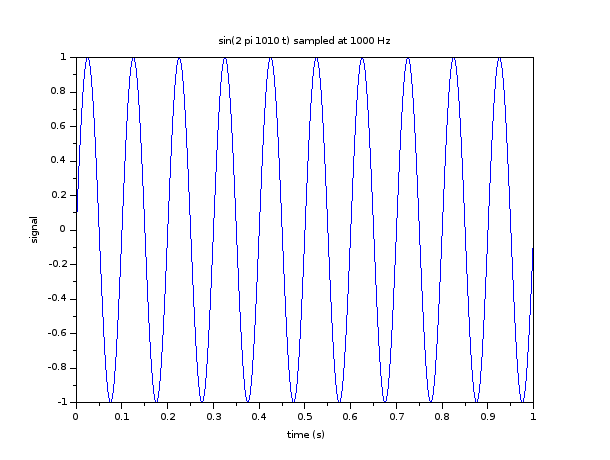When we sample a sine function at 300 Hz with a 1000 Hz sample rate, and we take a very very long sequence, then the calculated Fourier transform looks much like the actual Fourier transform of that sine function: a single peak at 300 Hz (we only consider positive frequencies, and take absolute values of the transform here):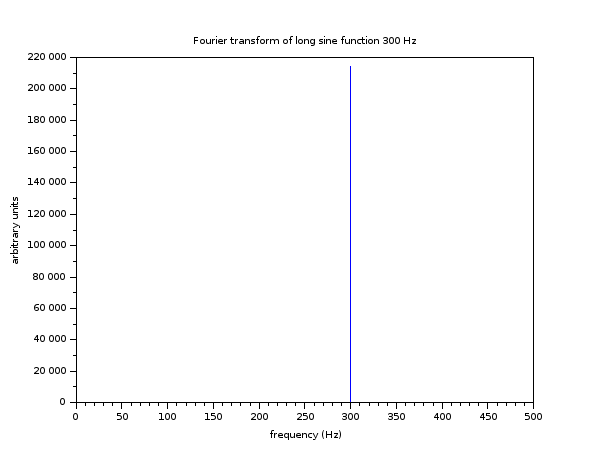However, if we now take a piece of sine function at 300 Hz over 131 ms, which will impose a grid of frequencies which are multiples of 7.63358... Hz, 300 Hz is not part of them, and leakage occurs: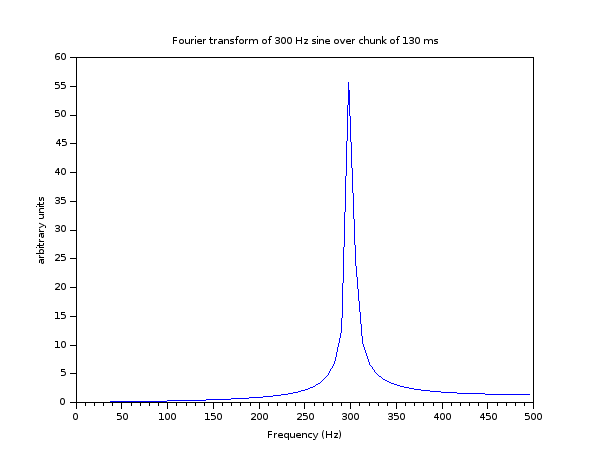If this time we take a piece of sine function at 300 Hz over 150 ms, the 300 Hz does fall on the grid of frequencies which are multiples of 6.6667 Hz, and the spectrum is correctly estimated again: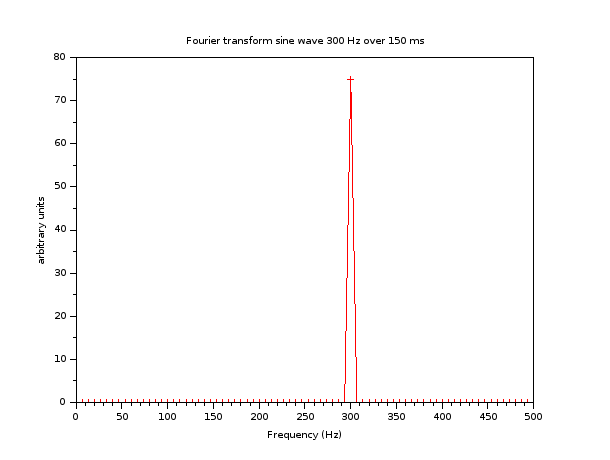Indeed, only one single spectral line on that grid, that of 300 Hz, is different from zero, as it should be for a pure sine signal.

We see again that the only way in which we can reliably calculate the Fourier transform from a finite piece of signal, is when the original signal is periodic, and when the finite piece that we take is exactly equal to a number of periods of the original signal.  In that case, we calculate in fact, the Fourier Series coefficients of that periodic signal.  This can be pretty annoying, because most of the time, the signal we're looking at is not periodic.  As such, the result will be vastly different for frequency components in the original signal which happen to be on the frequency grid of Fw and frequency components which happen not to lie on that grid.  The first will be represented faithfully, and the second will suffer from leakage.

We illustrate that with two spectra, both calculated with a sampling rate of 1 KHz, and with a time window of exactly 200 ms long, so that Fw equals 5 Hz.  We first take a signal composed of a sine at 300 Hz, and one at 310 Hz which fall on the grid.  We next do the same analysis, but on a signal composed of a sine at 302 Hz and one at 312 Hz (which miss the grid).  Note that the difference between both is each time 10 Hz.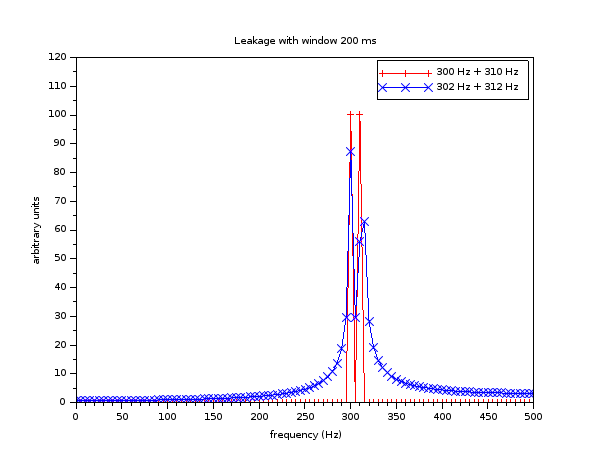The difference between the results of spectral calculation of these very similar signals is very strong.  As expected, the 300/310 Hz signal is perfectly represented, but the leakage effect of the 302/312 Hz signal is pretty nasty.   When we want to find out the spectral content of a signal of which we don't have any a priori periodic expectation, and which can in principle contain every continuous spectral contribution, it is annoying that certain frequencies are treated totally differently from others.  Indeed, when doing some spectral analysis, we might not make an a priori distinction between the possibility of having a signal consisting of a 300 Hz and a 310 Hz component, or a signal having a 302 Hz and a 312 Hz component.  The very fact of using a window of 200 ms simply means that we are interested in frequency resolutions of the order of 5 Hz.  So we don't really want a totally different kind of result for a 300/310 Hz signal, and a 302/312 Hz signal.

### Windowing functions

The strong difference between the 300/310 Hz signal and the 302/312 Hz signal in the previous example comes from the fact that a time window of 200 ms implies a frequency grid of 5 Hz step, and that periodic functions on that grid are faithfully represented, while other frequency components suffer leakage.  One might be interested in a technique which renders all frequency components "more equal for the law" so to say: we don't need perfect representation for those special frequencies on the grid, if this allows us to reduce the leakage of "outlaws" that don't fall on the grid.   This can be achieved partly by making the borders in time "fuzzy", by applying what is called a window function.

The standard window function we have taken up to now is the so-called rectangular window, which picks out a faithful copy of the time signal between 0 and T and puts everything to 0 outside.  The "everything to 0 outside" is not negotiable, but we could make the transition to 0 smoother around 0 and around T, by diminishing the amplitude of the signal near 0 and near T.   There are several window functions that have been proposed.  Window functions are somewhat like frequency filter approximations: they try to solve a mathematically impossible problem in one or another optimal way.   What one wants of a windowing function, is that its Fourier transform, which will give the spread of each frequency component in the original signal, has a narrow central lobe, and has no or very small side lobes.  Ideally, the Fourier transform of the window function would be a Dirac pulse... but then the window would be infinitely large in the time domain, and not limit the signal at all !  So a window function has the further constraint to be equal to 0 outside of the interval 0 to T seconds.

A non-exhaustive list of window functions:

• the rectangular window (standard window)
• triangular window
• Parzen (or de la Vallée Poussin) window
• Welch window
• Hamming window
• Hann window
• Blackman window
• Nuttal window
• Blackman-Harris window
• Flat top window
• Cosine window
• Gaussian window
• Tukey window
• Slepian window
• Kaiser window
• Dolph-Chebyshev window
• Lanczos
• ...

The right choice of window depends on the application at hand, requires some expertise, and in several cases, doesn't really matter much.  One only has to be aware of their effects and verify in how much these effects can influence the result for the application at hand.  In many cases, there's a trade-off between the width of the central lobe, and the intensity and roll-off of the side lobes in the Fourier transform of the window.

Let us illustrate this with two rather extreme cases: the rectangular window versus the Blackman window. The Blackman window looks like this: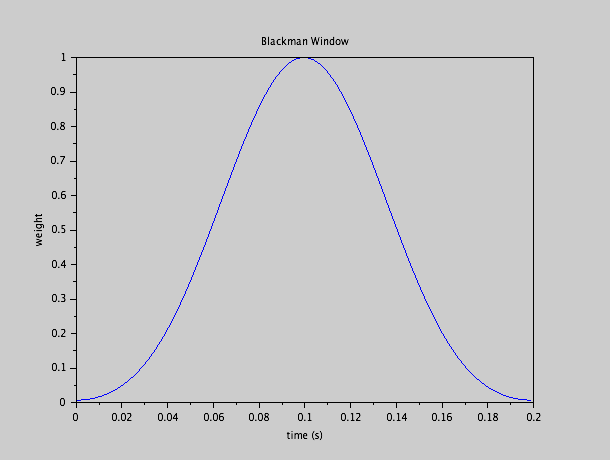We apply exactly the same conditions as with our illustration of a 300 Hz and 310 Hz signal, which was represented perfectly with the rectangular window, and the 302 Hz and 312 Hz signal, which had strong leakage, but this time, we apply a Blackman window.  Here are the estimated Fourier transforms: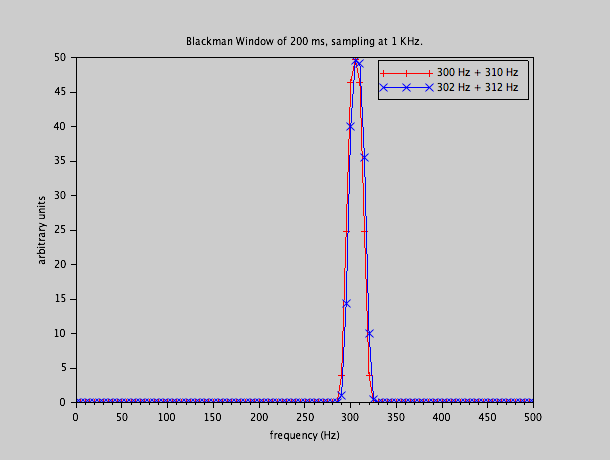The effect is striking !  There's almost no difference between the two cases, except that the 302/312 couple has a spectrum, shifted slightly to the right, as it should be.  There is almost no "far away" leakage as compared with the rectangular window.   But probably the most striking thing is that we don't have two peaks !  We cannot resolve the 300 Hz from the 310 Hz any more.

This is a usual trade-off: side lobes ("far away leakage") versus frequency resolution (the ability to distinguish two nearby frequency components).  The other trade-off consists in "all frequencies are equal for the law" versus "there are privileged frequencies on the grid, and horribly leaking frequencies off the grid".  Clearly, the rectangular window has very good frequency resolution, but horrible far-away leakage, and frequency Apartheid.  The Blackman window tends on the other side: bad frequency resolution (not able to distinguish 300 Hz from 310 Hz), but good far-away leakage (almost in-existent) and a high level of frequency-fairness.  This is why it takes some expertise to adapt the window choice to the application at hand. There is no universally better window than another, it depends on what the user wants to do with the spectrum.

### DFT, FFT and Fourier transformation

The Fast Fourier Transform is nothing else but an efficient algorithm to implement the Discrete Fourier Transform.  The DFT applies on a finite set of samples, that is, a windowed signal, limited from 0 to T, and sampled at a sample rate 2 fs so that there are N samples.

The Discrete Fourier Transform is nothing else but the calculation of the discrete lines of the actual Fourier Transform (reduced to a Fourier Series) of the repeated piece of signal into a reconstructed periodic signal on a grid of multiples of frequency Fw up to frequency fs.  The DFT are N complex numbers (the negative frequencies come in too, down to -fs).  If the Fourier transform of the repeated piece of signal has higher frequency components than fs then aliasing will occur (even if the original signal, before being windowed, didn't contain any frequency components above fs).  That is to say, the component of the DFT that comes out at, say, 7 Fw will be the sum of the sampled value of the Fourier transform of the single windowed piece of the signal at 7 Fw diminished with the sample value of that Fourier transform at 2 fs - 7 Fw, increased with the sample value at 2 fs + 7 Fw and so on.

It is the best estimate of the value of the Fourier transform at 7 Fw of the original signal that we can obtain, if we don't have any other information than the sampled data, but we know that it suffered aliasing and leakage.

There are some normalisation factors to be taken into account too to go from the N-point DFT to the estimate of the genuine continuous-time Fourier transform.

### The DFT as a Finite Fourier Series

This is the sole case where the DFT can give exact results: when we have a periodic input signal with period T, where we take one such period, and where we sample at a sample frequency 2fs such that in the real signal, no Fourier terms appear above fs. or at least, that we are willing to have these higher order terms, which are small enough, not matter if they alias.

Let us consider the 50% duty cycle function which is 1 half of the time, and 0 the rest of the time, over a period of 0.4 seconds.

The mathematical Fourier Series equals:

`f(t) = 0.5 + 2/π Σ 1/n sin(2 π t / T)  `

where the sum is over uneven n starting at 1.

The coefficients are, in other words:

```for n = 0: 0.5
for n = 1: 2/π = 0.6366...
for n = 3: 2/3π = 0.2122...
for n = 5: 2/π 1/5 = 0.1273...
for n = 7: 2/π 1/7 = 0.0909...```

etc...

If we make the sum over the first 5 non-zero terms in the series, we can see how this series will converge to the rectangular signal that we have been describing: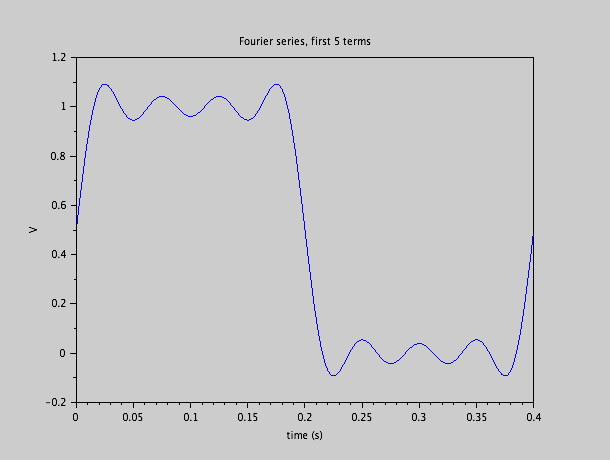Let us now sample one period of the rectangular signal, at different sampling rates, so that we have different numbers of samples: 400, 40 and 8: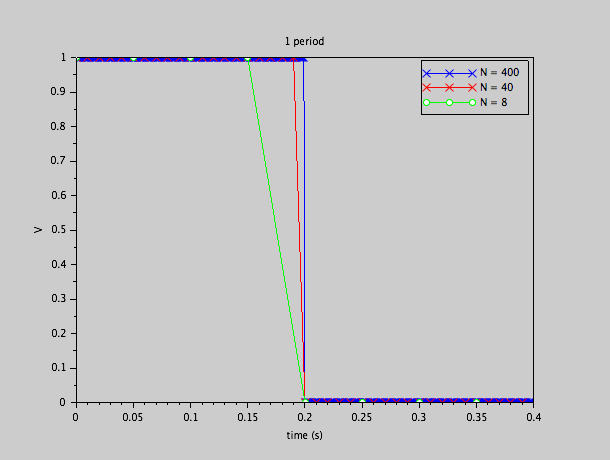We already see an effect: although the original function rises to 1 at t = 0 exactly, and drops to 0 at t = 0.2 exactly, the sampled values are such that the rise and drop occur somewhat earlier.  This will result in a small phase shift in our Fourier coefficients, as we will see.

The DFT of the series with 400 samples will also contain 400 coefficients, of course, of which the second half will be the complex conjugate of the first half.  As they are complex numbers, we will plot their absolute values: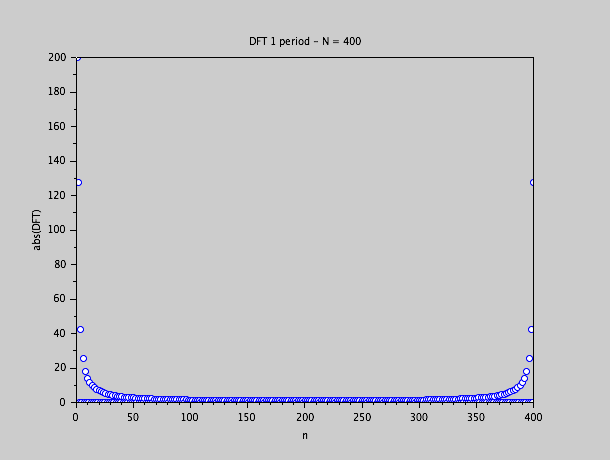The DFT values are:

```200
1 - 127.32 i
0
1 - 42.43 i
0
1 - 25.45 i
0
1 - 18.17 i```

...

The DFT values should be divided by N/2 to have the estimation of the Fourier coefficients in the way they are mathematically defined in the Fourier Series.  The real term is the coefficient of the cosine term, and minus the imaginary part is the coefficient of the sine term.   The very first term is the constant of the series.  This first term should in fact be divided by N and not by N/2.

If we divide by N/2 (here 200) and we take the absolute value, then we find:

```0.5
0.6366
0
0.2122
0
0.1274
0
0.0910```

...

As we see, these values are very close to the theoretical coefficients in the Fourier Series of the square function.  However, in the theoretical Fourier Series, there were only sine functions, and all the cosine terms were absent.  Here, we see that the complex DFT values have a slight real part (equal to 1 before division by N/2).  That would imply that there would be a small non-zero coefficient of the cosine terms too.  This is the result of the slight phase shift that we could see in the time plot: the fact that, because of the finite sampling, the signal starts rising before t = 0 and starts falling before t = 0.2.

Let us now look at what happens to the DFT when we use only 40 samples instead of 400: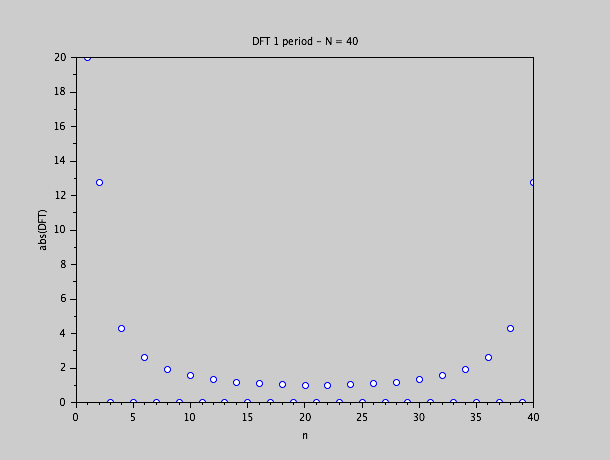```20
1 - 12.7062 i
0
1 - 4.1653 i
0
1 - 2.4142 i
0
1 - 1.6319 i```

...

We already notice that relatively speaking, the phase shift is more important towards the real (cosine) part: the real part is still 1, but the absolute values are about 10 times smaller, so the phase shift is 10 times larger as was the case with the 400 samples.

If again, we take the absolute value, divide by N/2 = 20 (except for the first term which should be divided by N = 40), we obtain:

```0.5
0.6373
0
0.2142
0
0.1307
0
0.0957```

...

The values still look all right at first sight.... except that they are now somewhat higher than the theoretical values, especially the higher order terms.  For instance, the coefficient of the 7th harmonic is theoretically 0.0909..., and the 400 point DFT gave us 0.0910.  The 40 point DFT gives us 0.0957.  We start seeing the effect of aliasing.

The effect will even be more pronounced if we only take 8 sample points: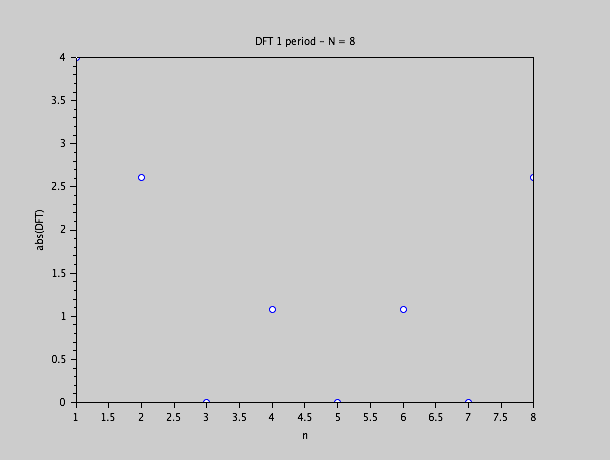The whole DFT is this:

```4
1 - 2.414 i
0
1 - 0.4142 i
0
1 + 0.4142 i
0
1 + 2.414 i```

Here, we see that the 4 last points are the complex conjugate values, so they don't learn us anything that isn't present in the first 4 values.  The phase shift is now dramatic: the cosine terms are of the same order as the sine terms.

The absolute values and normalisation by N/2 give us the following for the first 4 terms:

```0.5
0.6533
0
0.2706```

As we can see, the alias effect is pretty strong and even visible on the first sine term: we find 0.6533 instead of the theoretical value of 0.6366.

We can conclude that the DFT allows us to calculate the Fourier Series coefficients of a genuine periodic function, but that the finite sampling can introduce a phase shift, and introduces aliasing if the original Fourier Series doesn't cut off before the Nyquist frequency.  We also notice that the normalisation is only dependent on the number of points, N, in the DFT, and that the time scale doesn't enter.

Finally, we illustrate what happens if we take a periodic signal of which we take a window that is an integer multiple of the signal period.  We take our same square function, but this time we take a window that is 5 times the period (that is to say, 2 seconds, instead of 0.4 seconds which is the signal period).  We will sample with N = 2000, N = 200 and N = 20.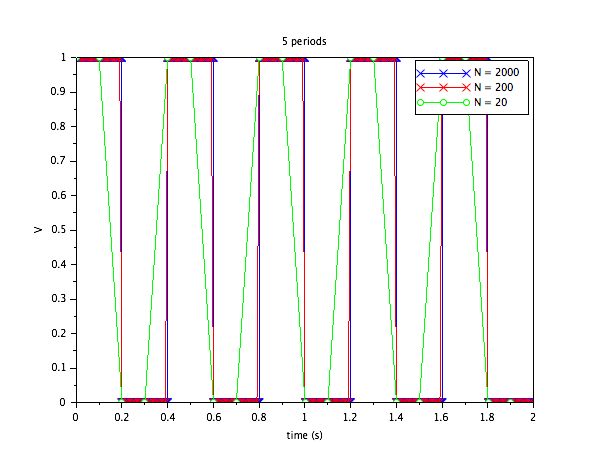In this repeated plot, one sees better the phase shift "to the left" when the number of samples decreases: the green signal clearly has its pulses "earlier" than the red and the blue signals ; if one pays careful attention, one even sees that the red curve is slightly more to the left than the blue one.

The DFT values (their absolute value) of the case of 2000 samples is shown next: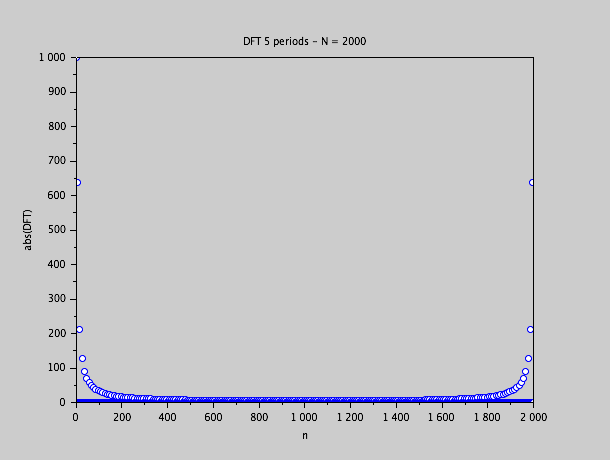We see that they take on 5 times larger values than in the case of one single period and 400 samples, which corresponds to the same sample frequency (5 times more time covered, and 5 times more samples); this is normal because we will also have to divide by N/2 which is this time not 200, but 1000 (5 times larger).

The case of N = 200: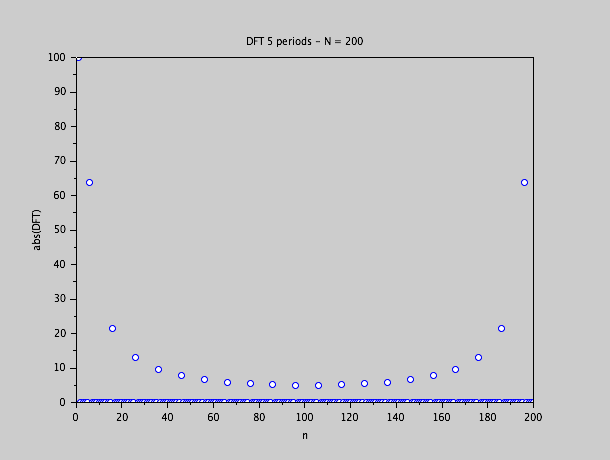shows more clearly the following fact: between each non-zero DFT value, there are 9 zero values (there used to be only one).  This is normal, because Fw now has 1/5 of the value (because we consider a time window which is 5 times longer).  However, the frequency components in the Fourier Series haven't changed their frequencies.  There are simply 4 sine (and cosine) terms which have coefficient 0 before we reach another frequency which is present in the original series.

Indeed, when the window was equal to the period, that is, 0.4 seconds, Fw was 2.5 Hz.  In the Fourier Series, there is a term at 2.5 Hz, there is a term at 5 Hz (which happens, for the square function, to be zero), there is a term at 7.5 Hz, there is a term at 10 Hz (which happens to be zero) and so on.  But now, the period is 2 seconds, which means that Fw is now 0.5 Hz.  So the DFT calculates the contributions at 0.5 Hz, at 1 Hz, at 1.5 Hz, at 2 Hz, and at 2.5 Hz.  But only this last term is present in the Fourier Series of our square signal (which hasn't changed of course, because the signal is still the same).  So in between each original term (2.5 Hz, 5 Hz, 7.5 Hz etc...) there are now 4 new DFT values, spaced by 0.5 Hz.  But these are not present in the Fourier Series, and hence will come out to 0.

As moreover, every other Fourier Series term is 0 in our particular case of a square wave, this means that there will be 9 zero values in the DFT, between each couple of non-zero values (except in the beginning).

The first few values of our 200 point DFT are:

```100
0
0
0
0
5 - 63.5310 i
0
0
0
0
0
0
0
0
0
5 - 20.8265 i
0
0```

...

Normalizing by N/2 and omitting the 4 extra zeros, we find, in absolute value:

```0.5
0.6373
0
0.2142
0```

...

Note that these values deviate somewhat from the theoretical values (due to aliasing), but are strictly identical to the case of 1 single period and N = 40.  This is important to notice: the case of one period and N = 40 is perfectly equivalent to the case of 5 periods and N = 200, except for the fact that we add 4 useless zeros between each value, and that we have to divide by 100 instead of by 20 to recover the Fourier Series coefficients.

### The DFT and the Fourier Transform

We now know that the DFT is a way to calculate numbers which are exactly, or approximately, the coefficients of a Fourier Series, after a normalisation by N/2.  But what about the Fourier Transform ?

First of all, there is no doubt about the convention for Fourier Series, but there are different conventions for the Fourier Transform (essentially, depending on whether one is a mathematician, a physicist or an electrical engineer).  We will use the convention that is normal for electrical engineers:

F(ω) = ∫ f(t) e-jωt dt

where ω is the angular frequency.

In that case, we already know that the DFT values are proportional to the samples of F(2 π n Fw) where F is the Fourier Transform of the finite piece of signal from 0 to T, and put to 0 everywhere outside of that interval.  What is that proportionality constant ?

It is easy to show that the n-th complex Fourier Series coefficient equals cn = 1/T X(2 π n Fw), where T is the length of the finite piece of signal from which 1) we took the Fourier Transform X, and 2) which we repeated into a periodic signal with period T.

But the cosine and sine coefficients are respectively twice the real part and minus twice the imaginary part of cn which themselves are 2/N * DFT.

In other words, cn = 1/N * DFT(n + 1) at least for n < N/2, from which we deduce:

X(2 π n Fw) = T/N * DFT(n + 1) for n < N/2

The estimation of the complex value of the Fourier Transform of the finite piece of signal from 0 to T at frequency n Fw and hence angular frequency 2 π n Fw is given by the (n + 1)th value of the DFT, multiplied with T/N, which is nothing else but the sampling period.

Let us verify this with our same piece of signal, namely the signal that is 1 between t = 0 and t = 0.2 s, but is zero everywhere else.  This time we take a long T, say, 5 seconds.  That will sample the Fourier transform every 0.1 Hz.

The Fourier Transform of the piece of signal which is 1 between t = 0 and t = 0.2 s, and zero everywhere else, equals:

`F(ω) = i / ω ( cos(0.2ω) - i sin(0.2ω) - 1)`

as can easily be verified by a simple integration.

If we sample our function at a frequency of 500 Hz, then we will have N = 2500 samples, of which 100 are 1 and all the rest 0.  Mind you that this time, we don't make a periodic signal out of our original piece of square signal.  Given that we took a signal window T = 5s, the DFT will produce samples of the Fourier transform on a grid of 0.2 Hz, or 1.256 rad/s.  We compare the absolute values of the FT and these samples, reweighted with 0.002 s (the sample period), on a piece of the omega axis: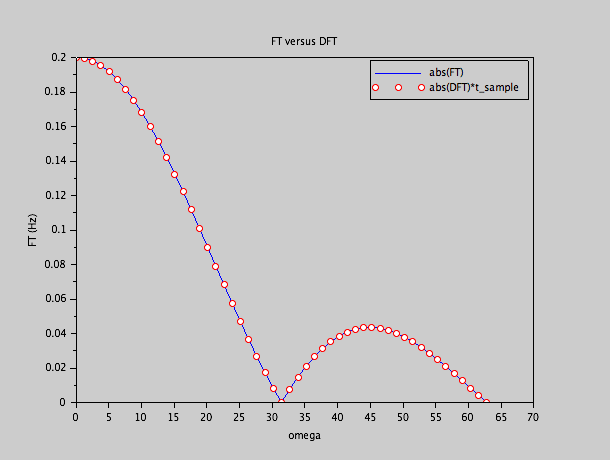We can compare the complex values too.  They work out well.  We may think that we were over-zealous when sampling at 500 Hz.  After all, we only wanted a plot up to 10 Hz (62.5 rad/s).  So a sample frequency of 20 Hz would be OK at first sight.... However, if we take lower sample frequencies, aliasing occurs and the complex values don't compare very will any more.  In the following plot, we did exactly the same as in the previous one, except that the sample frequency is now 25 Hz.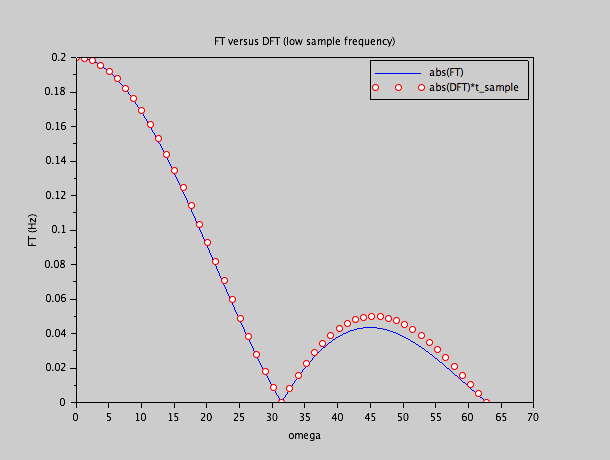We note the deviation between the samples and the true Fourier Transform.

### Conclusion

If we want to calculate numerically a Fourier Transform or a Fourier Series, the tool at hand is the DFT, which transforms a finite set of samples at a finite sample frequency and a finite time window, into a finite set of complex numbers.   These complex numbers can, if we consider our finite piece of signal a period of a periodic signal, be transformed into estimates of the Fourier Series coefficients of this periodic signal.  They can also, if we consider our finite piece of signal as such, be transformed into samples of the complex Fourier Transform of that piece of signal.

In all these cases, aliasing can occur, which is the unavoidable effect of using a finite sample frequency.

In as much as our finite piece of signal is to be representative for an infinite-duration signal, leakage will occur too except in very special cases.  This leakage is depending on the windowing function that one applies to "pick out a finite piece" of the signal.

In this contribution, we only talked about the estimation of a Fourier Transform or Fourier Series on a specific, deterministic, signal, and not about ensembles of signals.

The choice of the sample frequency, the period, and the window require expertise to match the resulting spectral estimation to the needs of the application at hand.  There is no universal solution that performs a perfect Fourier Transform, because we have to have a finite number of samples taken at a finite sampling frequency in the digital domain.